# Chapter 26: Class Coding Basics¶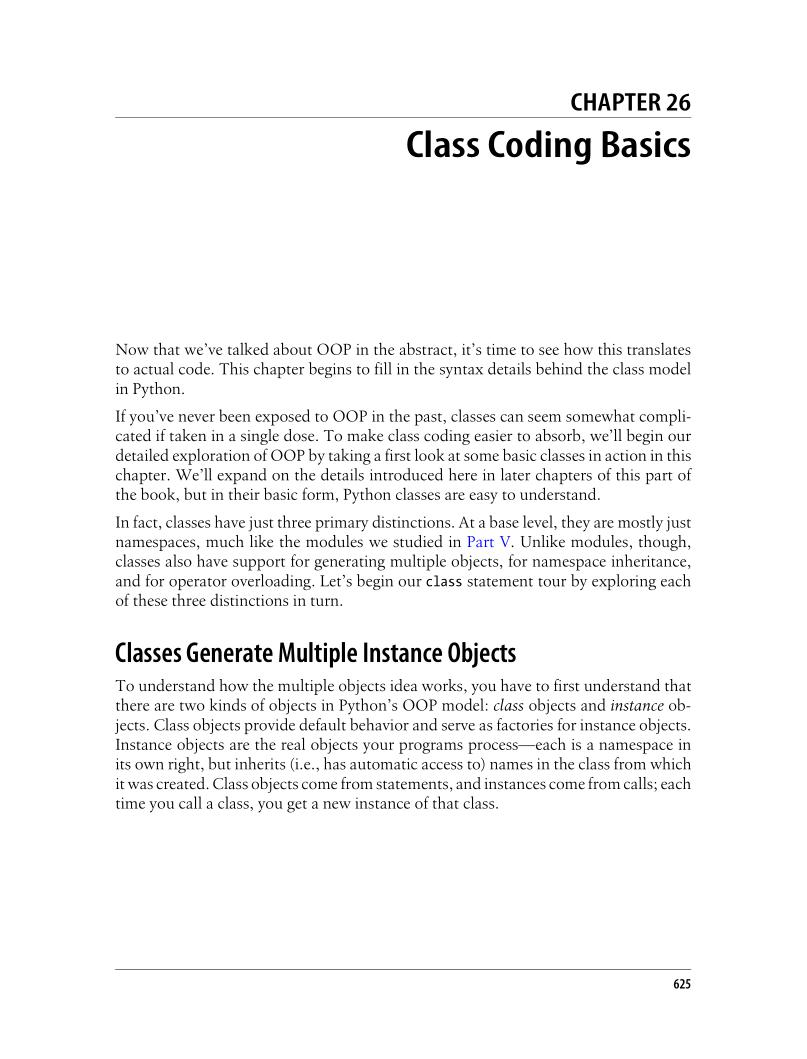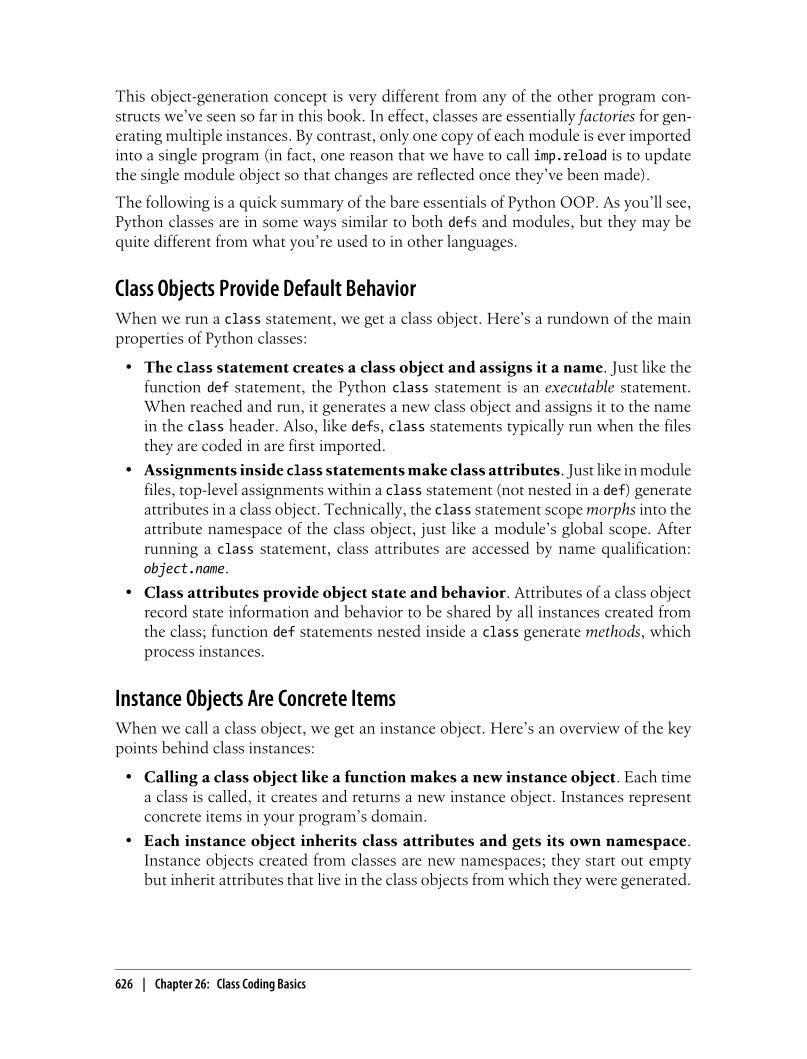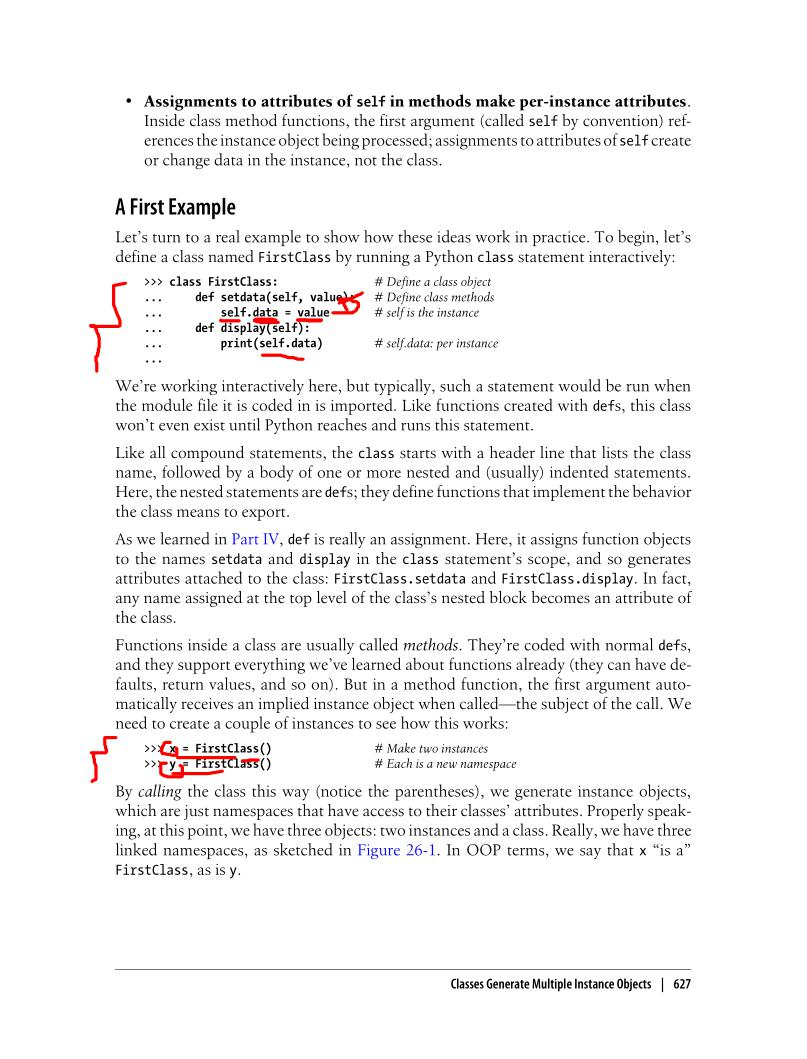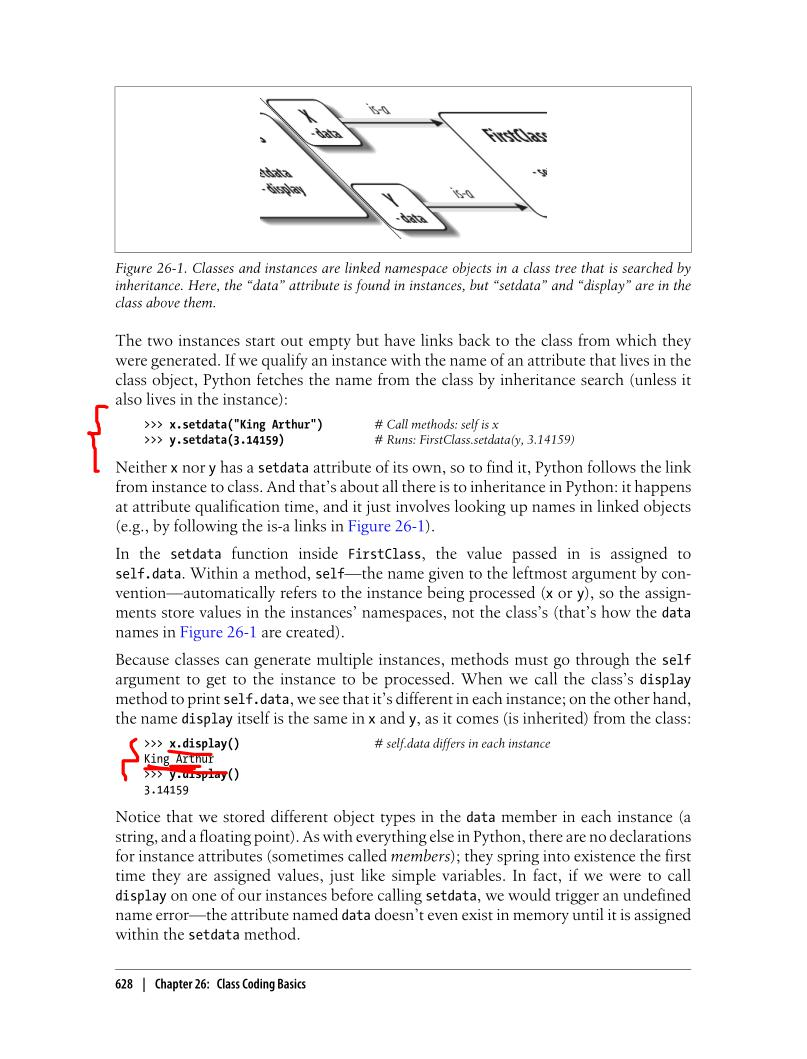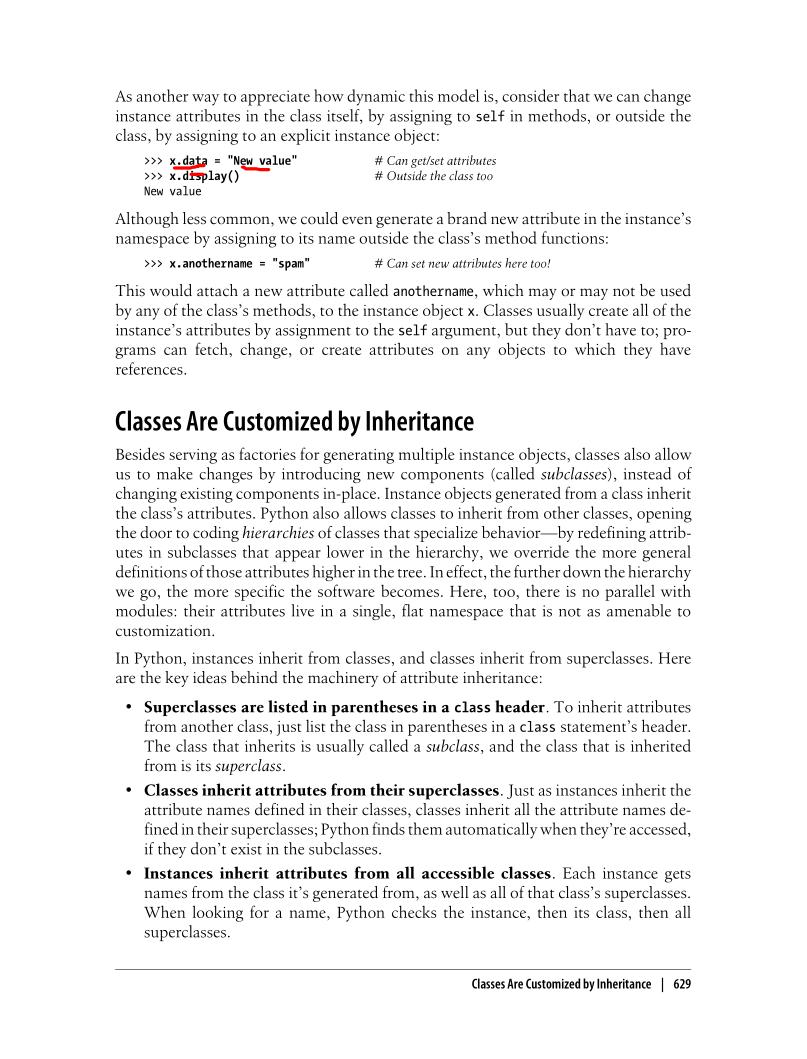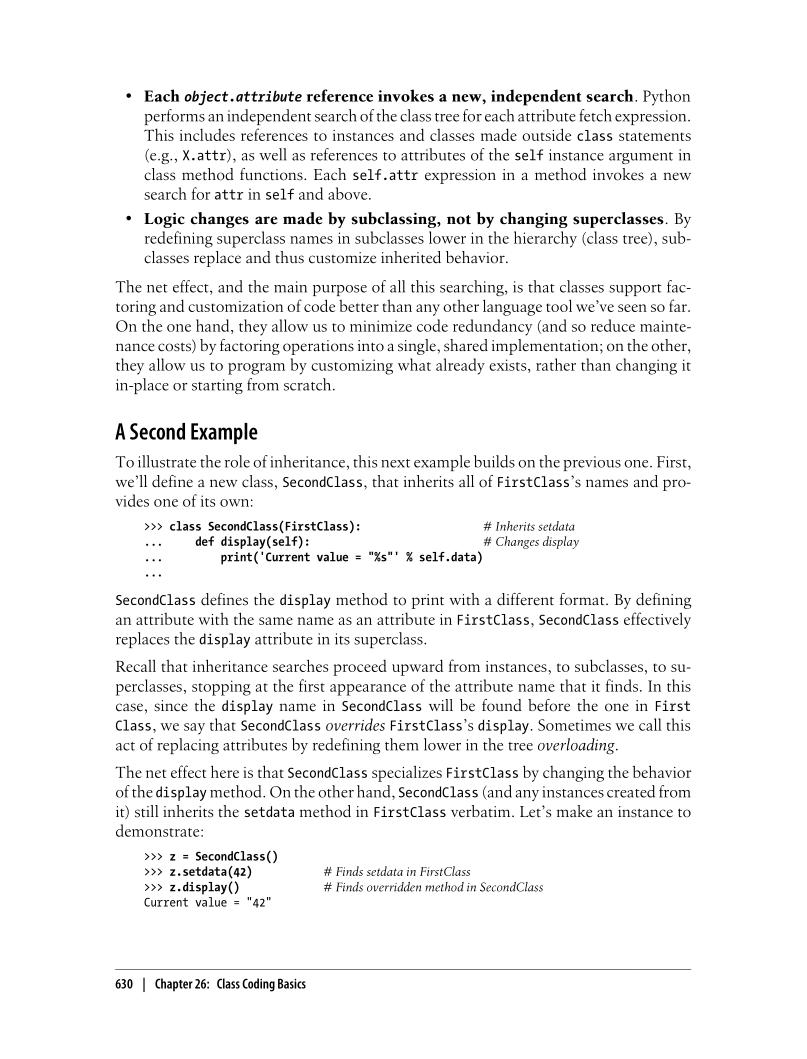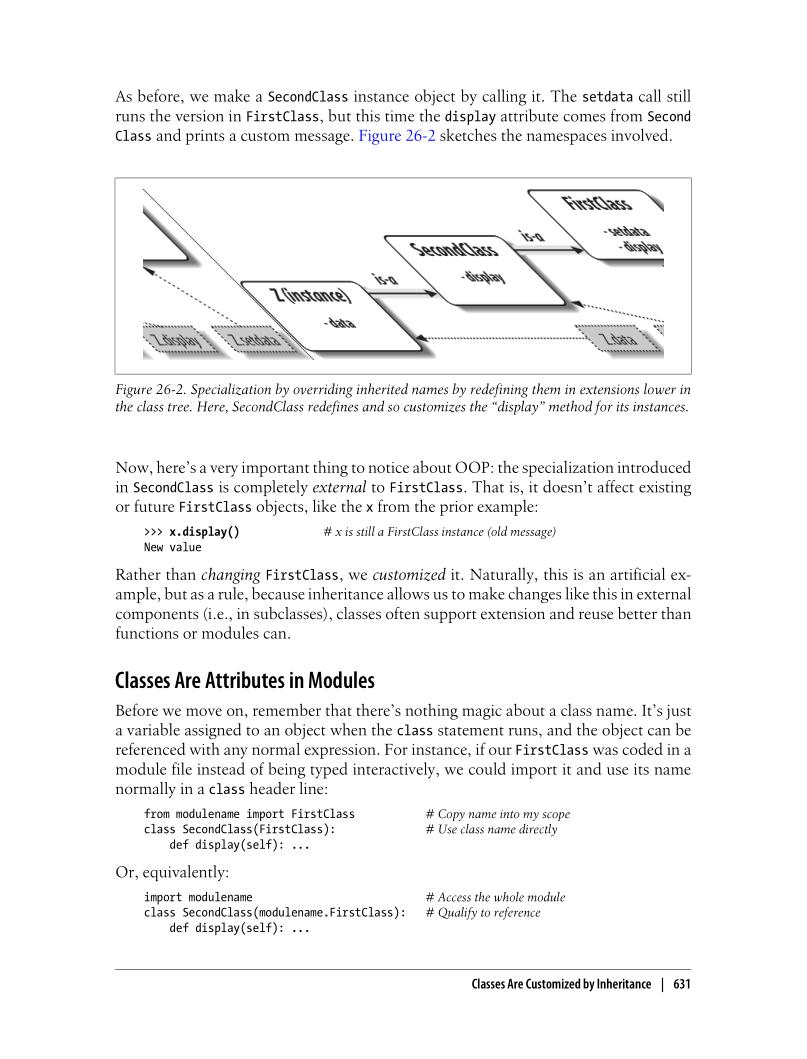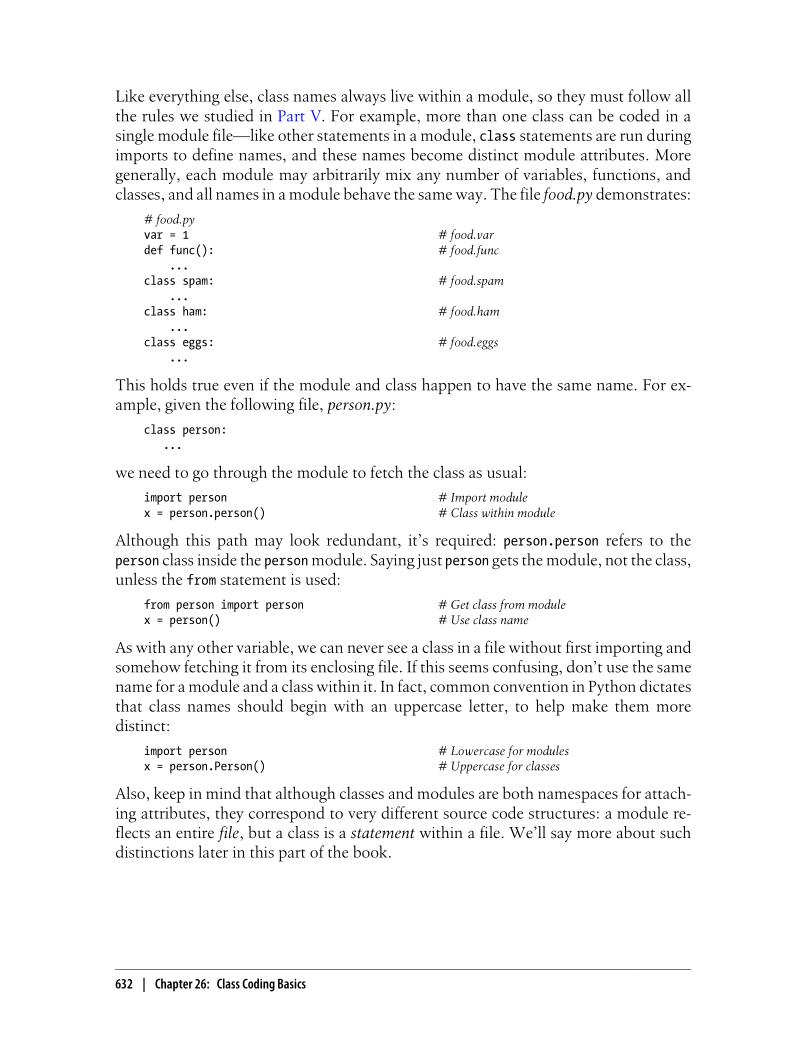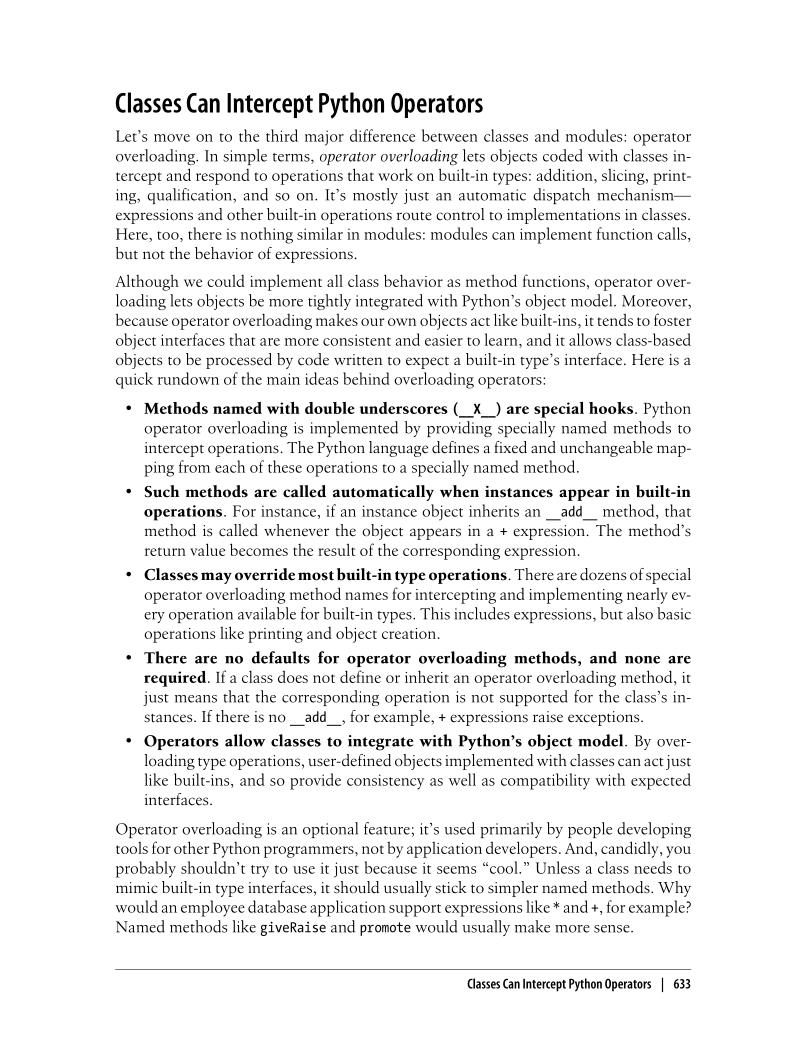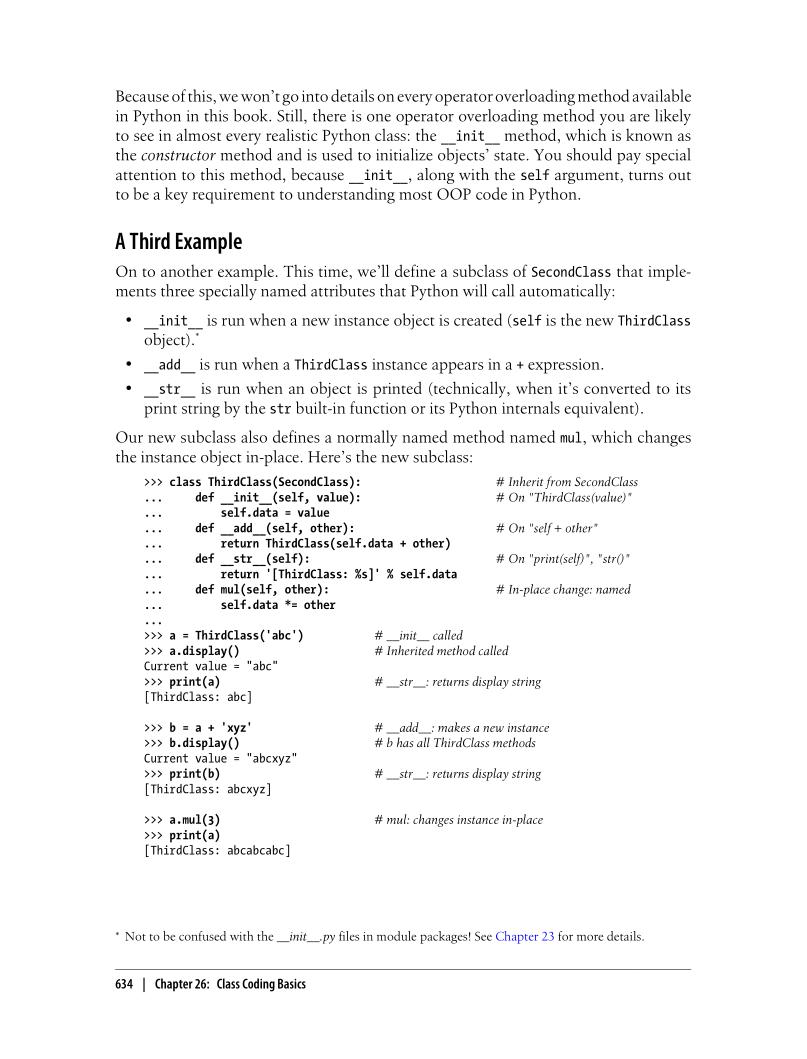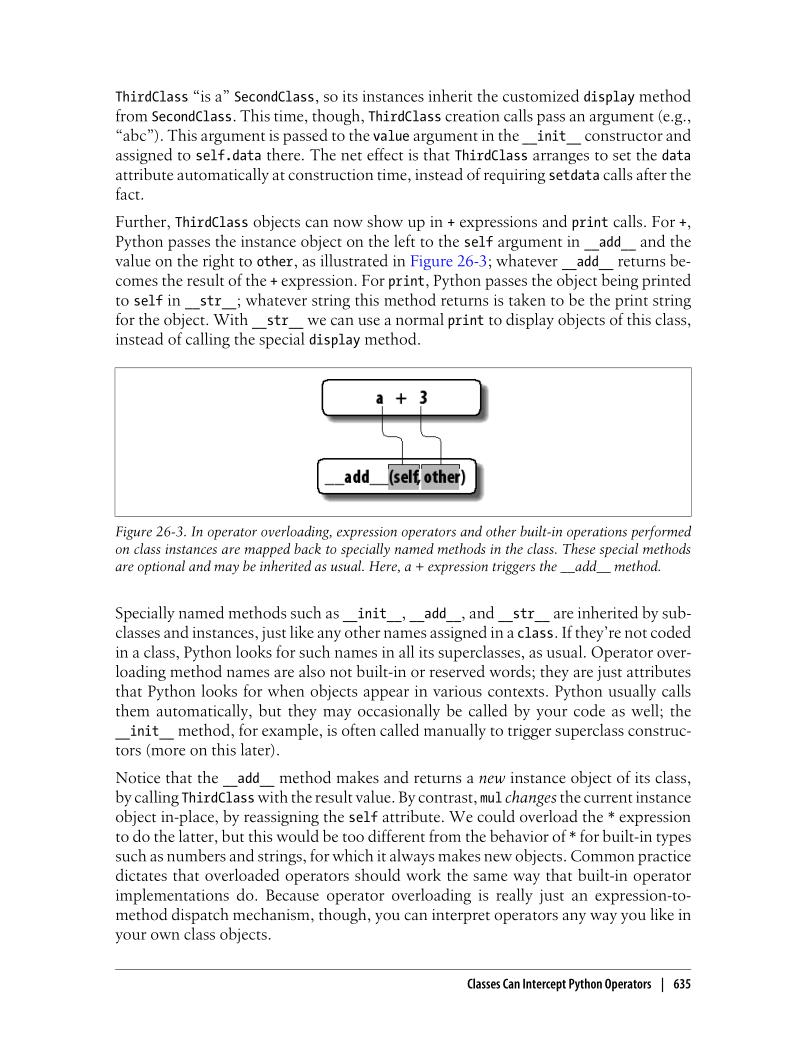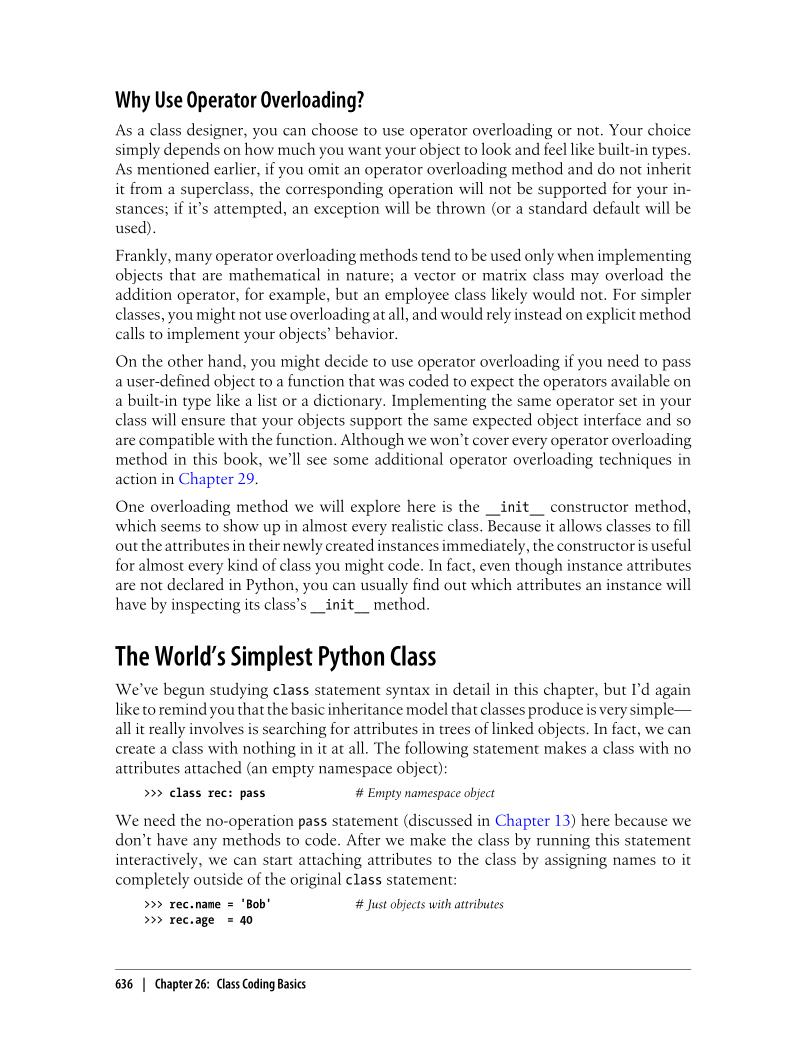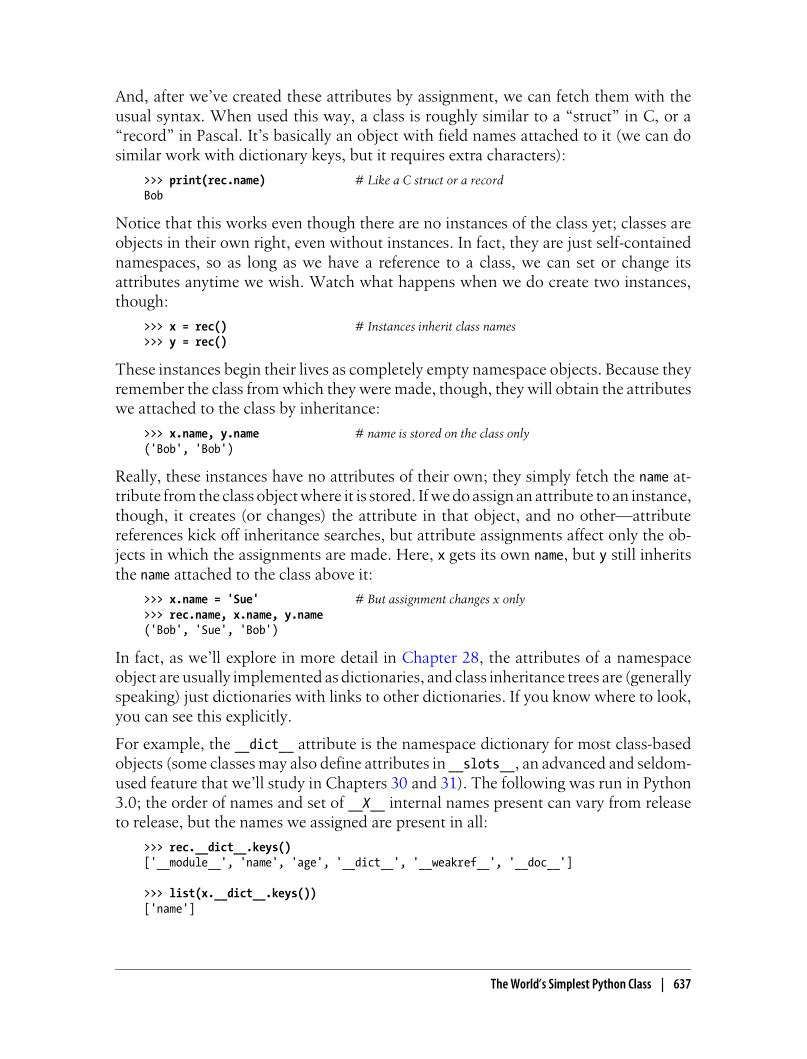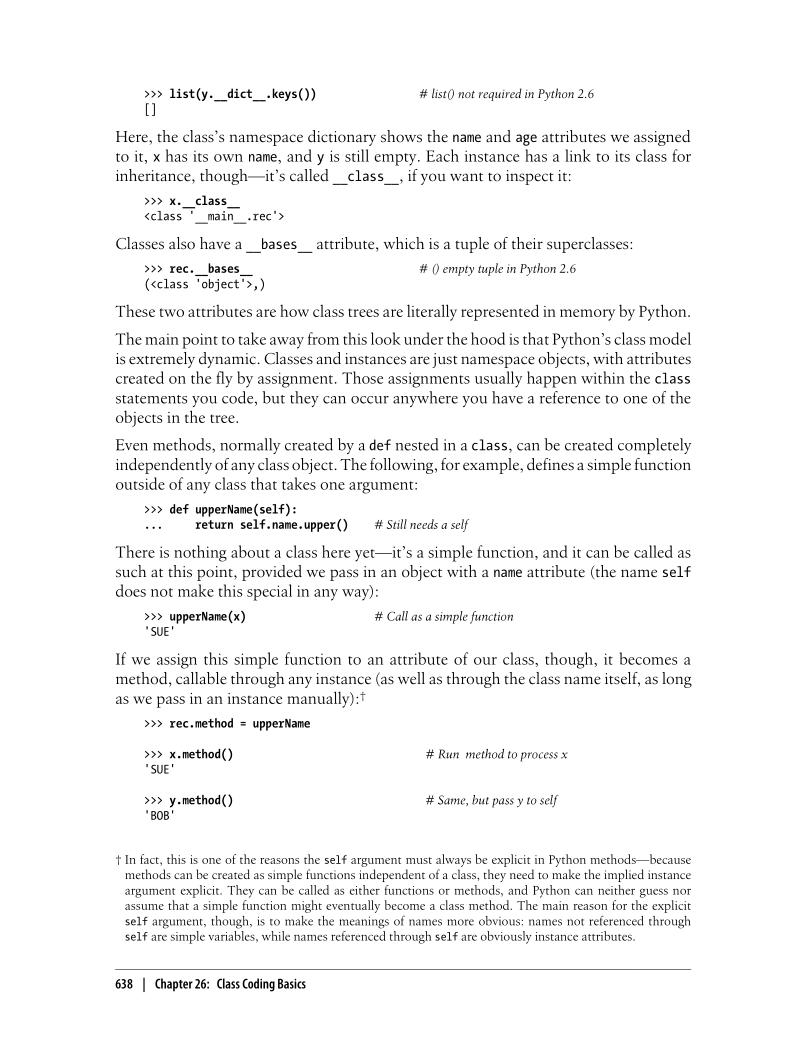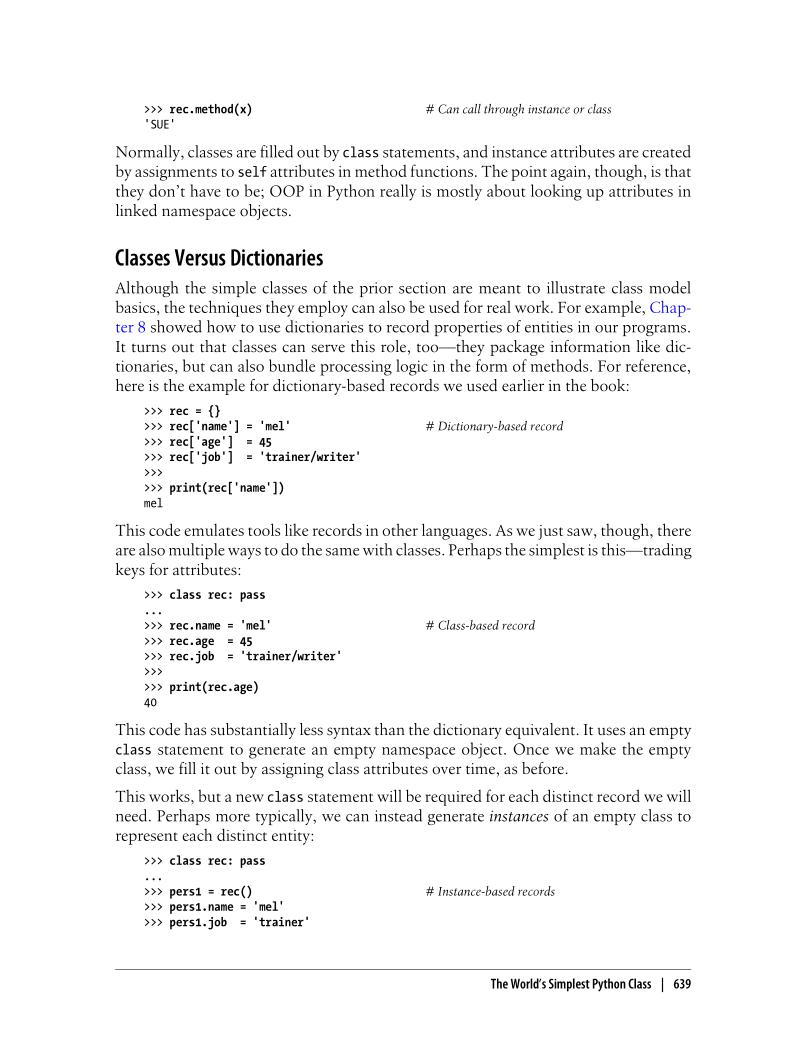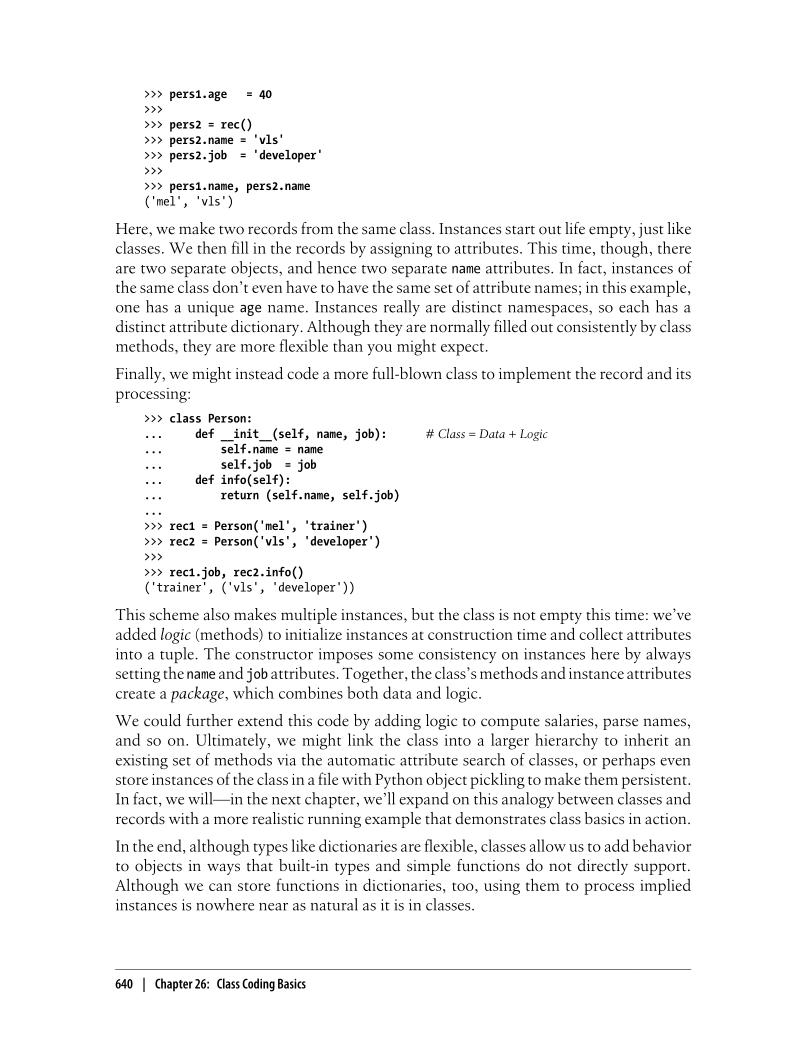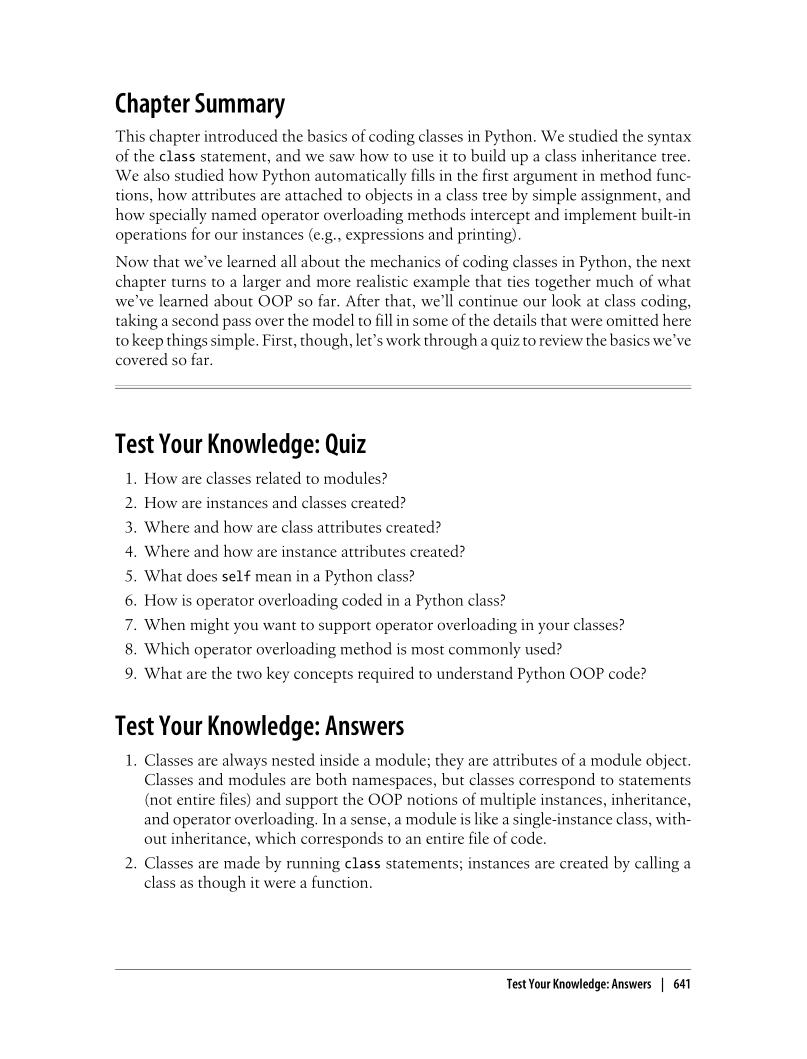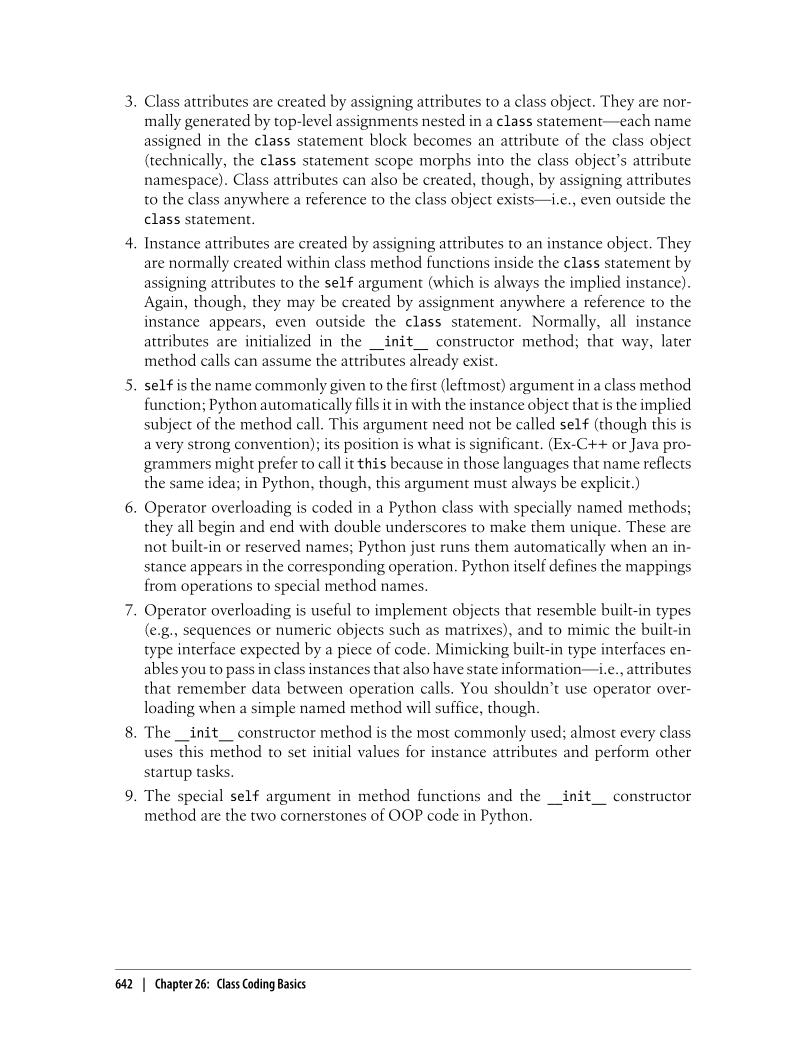```>>> class FirstClass:             # Define a class object
...   def setdata(self,value):    # Define class methods
...      self.data = value        # self is the instance
...   def display(self):
...      print(self.data)         # self.data: per instance

>>> x = FirstClass()              # Make two instances
>>> y = FirstClass()              # Each is a new namespace

>>> x.setdata("King Arthur")      # Call methods: self is x
>>> y.setdata(3.14159)            # Runs: FirstClass setdata(y,3.14159)

>>> x.display()
King Arthur
>>> y.display()
3.14159
>>> x.data = "New value"
>>> y.display()
New value

>>> x.anothername = "spam"
```
 ``` 1 2 3 4 5 6 7 8 9 10 11 12 13 14 15 16 17 18 19 20 21 22 23 24 25 26 27 28 29 30 31 32 33 34 35 36 37 38 39 40 41 42 43 44 45 46 47 48 49 50 51 52 53 54 55 56 57 58 59 60 61 62 63 64 65 66 67 68 69 70 71 72 73 74 75 76 77 78 79 80 81 82 83 84 85 86 87 88 89 90 91 92 93 94 95 96 97 98 99 100 101 102 103 104 105 106 107 108 109 110 111 112 113 114 115 116 117 118 119 120 121 122 123 124 125 126 127 128 129 130 131 132 133 134 135 136 137 138 139 140 141 142 143 144 145 146 147 148 149 150 151 152 153 154 155 156 157 158 159 160 161 162 163 164 165 166 167 168 169 170 171 172 173 174 175 176 177 178 179 180 181 182 183 184 185 186 187 188 189 190 191 192 193 194 195 196 197 198 199 200 201 202 203 204 205 206 207 208 209 210 211 212 213 214 215 216 217 218 219 220 221 222 223 224 225 226 227 228 229 230 231 232 233 234 235 236 237 238 239 240 241 242 243 244 245 246 247 248 249 250 251 252 253 254 255 256 257 258 259 260 261 262 263 264 265``` ```class A: """ Namespaces: The Whole Story:: 1. qualified and unqualified names are treated differently, and that some scopes serve to initialize object namespaces; 2. Unqualified names (e.g., X) deal with scopes; 3. Qualified attribute names (e.g., object.x) use object namespaces; 4. Some scopes initialize object namespaces (for modules and classes). Simple Names: Global Unless Assigned Unqualified simple names follow the LEGB lexical scoping rule outlined for functions in Chapter 17. 1. Assignment (X=value) Makes names local: creates or changes the name X in the current local scope, unless declared global; 2. Reference(X) Looks for the name X in the current local scope, then any and all enclosing functions, then the current global scope, then the built-in scope. """ def __init__(self, x=1, y=2, z=3): self.x = x self.y = y def __add__(self, other): return (self.x + other.x, self.y+other.y) def __repr__(self): return "%s, %s"%(self.x, self.y) class B: def __init__(self, x=10, y=20, z=30): self.x = x self.y = y self.z = z def __add__(self, other): return (self.x+other.x, self.y + other.y) def __repr__(self): return "%s, %s"%(self.x, self.y) class C(A,B): def __repr__(self): return "%s, %s"%(self.x, self.y) class D(B,A): def __repr__(self): return "%s, %s"%(self.x, self.y) class E(A,B): """ Where does an inheritance search look for an attribute? 1. start from an attribute first in the instance object; 2. in the class "def __init__(self, x, y)" 3. in all higher superclasses "def __init__(self, x, y)", from the bottom to the top of the object tree, and from left to right (by default), 4. from local class domain; 5. from all higher superclasses domain; """ #def __init__(self, x=100, y=200): # self.x = x # self.y = y x = 1000 y = 2000 z = 3000 def __repr__(self): return "%s, %s, %s"%(self.x, self.y, self.z) a = A() b = B() c = C() d = D() a0 = A(3,3) e = E() #e.x = 10000 print(a,b,c,d, a+a0, e, e.x, sep=" | ") print("-------------") print(d.__dict__) print(D.__dict__) print(e.__dict__) print(E.__dict__) print("------------") print(dir(e)) print(e.__doc__) # manynames.py X = 11 def f(): """ Access global X(11) # ohterfile.py >>> import manynames >>> X = 66 >>> print(X) 66 # 66: the global here >>> print(manynames.X) 11 # 11: globals become attributes after imports >>> manynames.f() 11 # 11: manynames's X, not the one here! >>> manynames.g() 22 # 22: local in other file's function >>> print(manynames.C.X) 33 # 33: attribute of class in other module >>> print(I.X) 33 # 33: still from class here >>> I.m() >>> print(I.X) 55 # 55: now from instance! """ print(X) def g(): """ Local (function) variable (X, hides module X) """ X = 22 print(X) class C: X = 33 """ Class Attribute (C.X) """ def m(self): X = 44 self.X = 55 X = 11 def g1(): print(X) def g2(): global X X = 22 print(X) def h1(): X = 33 def nested(): print(X) nested() print(X) def h2(): """ Finally, as we learned in Chapter 17, it's also possible for a function to change names outside itself, with global and (in Python 3.0) nonlocal statements--these statements provide write access, but also modify assignment's namespace binding rules """ X = 33 def nested(): nonlocal X X += 1 print(X) nested() print(X) if __name__ == "__main__": print(X) f() g() print(X) obj = C() print(obj.X) obj.m() print(obj.X) print(C.X) print(dir(g)) print(g.__globals__) print("----------------") g1();g2();h1();h2() print(E.__dict__) """ Namespace Dictionaries In Chapter 22, we learned that module namespaces are actually implemented as dictionaries and exposed with the built-in __dict__ attribute. The same holds for class and instance objects: attribute qualification is really a dictionary indexing operation internally, and attribute inheritance is just a matter of searching linked dictionaries. """ class super: def hello(self): self.data1 = "spam" class sub(super): def hola(self): self.data2 = "eggs" X = sub() print(X.__dict__) # Instance namespace dict print(X.__class__) # Class of instance print(sub.__bases__) # Superclasses of class print(super.__bases__) # object Y = sub() X.hello() print(X.__dict__) X.hola() print(X.__dict__) print(sub.__dict__.keys()) print(super.__dict__.keys()) print(Y.__dict__) print(X.hello) #print(X.__dict__["hello"]) """ Because attribute fetch qualification also performs an inheritance search, it can access attributes that namespace dictionary indexing cannot. The inherited attribute X.hello, """ print(X.__dict__, Y.__dict__) print(list(X.__dict__.keys())) print(dir(X)) print(dir(sub)) print(dir(super)) # classtree.py """ Climb inheritance trees using namespace links, displaying higher superclasses with indentation """ def classtree(cla, indent): print("."*indent+cla.__name__) # print class name here for supercls in cla.__bases__: # recur to all superclasses classtree(supercls, indent+3) # may visit super > once def instancetree(inst): print("Tree of %s" % inst) classtree(inst.__class__,3) def selftest(): class A: pass class B(A): pass class C(A): pass class D(B,C): pass class E: pass class F(D,E): pass instancetree(B()) instancetree(F()) class FirstClass: # Define a class object def setdata(self, value): # Define class methods self.data = value # self is the instance def display(self): print(self.data) # self.data: per instance print("+++++++++++++++++++++++++++++++++++++++") print(FirstClass.__dict__) print("+++++++++++++++++++++++++++++++++++++++") def FirstFunction(value): data = 0 def setdata(value): nonlocal data data = value return data def display(): print(data) setdata(value) display() if __name__ == "__main__": selftest(); FirstFunction(3) x = FirstClass() y = FirstClass() x.setdata("King Arthur") print(x.data) y.setdata(3.14159) #y.data = 951413 print(y.data, y.__dict__, FirstClass.__dict__) class C: count = 0 a = C() b = C() c = C() c.count += 10 C.count += 100 print(a.count, b.count, c.count) print(a.__dict__, b.__dict__, c.__dict__, C.__dict__) ```